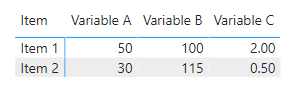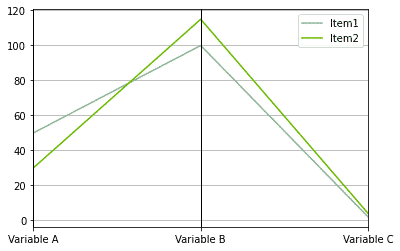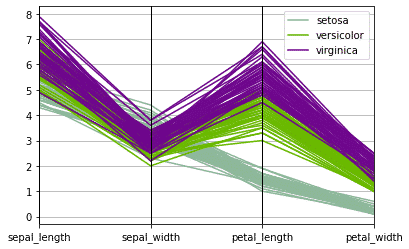# Parallel Coordinate Plots

Multivariate numerical data can be visualized using a parallel coordinates plot. This visual compare samples or observations across several numerical variables.

The X- axis is used to represent each feature or variable. All of the axes are parallel to one another and equally spaced apart. A distinct scale and unit of measurement can be used for each Y-axis.
Then a connection is created horizontally across each variable.

Although this is a great plot for multivariate data, if there are many data points to be plotted, the parallel coordinates chart can become highly congested. To prevent cluttering the graphic, you should only emphasize a select few points. We’ll cover how to plot a parallel coordinates chart in Python. Other alternatives to this plot are radar charts, which organize parallel lines (axes) radially.### Let’s Visualize Data with the Parallel Coordinate Plot

This is a small dataset that will help you understand what this plot can achieve. The best way to think about the data is that each column is a variable of data and this data can be divided by a dimension or a class.

``````#import plotly, pandas and parrallel cordinates
import pandas as pd
from pandas.plotting import parallel_coordinates
#create a small dataframe
small_data =  pd.DataFrame(
{'Item':['Item1','Item2'],'Variable A':[50,30],'Variable B':[100,115],\
'Variable C':[2,4]})
parallel_coordinates(small_data,class_column='Item')
``````Now, this is a very easy data structure to visualize as a parallel coordinate plot. We can see that the class or dimension creates the color variation and the data for each variable are connected by the item class. The diagonal/horizontal line just connects where the points exist on the Y-axis thus creating a relationship.

Let’s create a more complex plot using the iris dataset that can be found in seaborn

``````#load the visualization library for your plot
from pandas.plotting import parallel_coordinates
parallel_coordinates(data,'species')``````• in
• |
• November 17, 2022#### Gaelim Holland

Subscribe
Notify of